# Mathematics of General Relativity

Back to General Relativity

#### Differential Equations

• Differential equations are magic:
• Differential equations enable the instant-by-instant calculation of the state of a physical system from an initial time to any future time.
• A differential equation is an equation with derivatives.
• A derivative is the rate of change of a function relative to a variable.  For example:
• Velocity is the rate of change of location relative to time.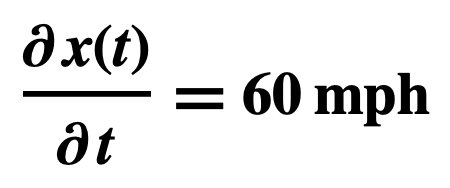• Acceleration is the rate of change of velocity relative to time.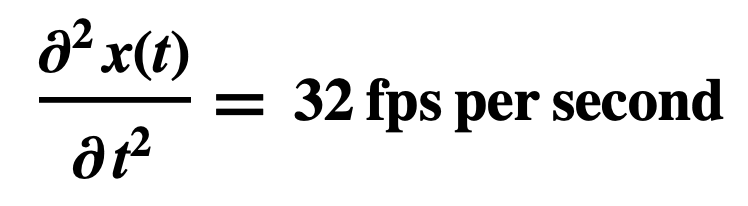• How differential equations are solved:
• Analytic Methods, by manipulating formulas
• Numerical Analysis, by crunching numbers
• britannica.com/science/differential-equation
• Differential equations are very common in science and engineering, as well as in many other fields of quantitative study, because what can be directly observed and measured for systems undergoing changes are their rates of change.
• The solution of a differential equation produces a function that can be used to predict the behavior of the original system, at least within certain constraints
• How Newton’s Equation of Motion works: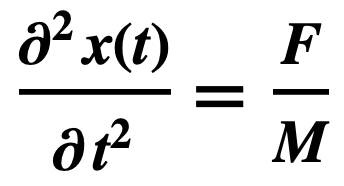• You first determine a particle’s acceleration by dividing the net force F on it by its mass M, e.g.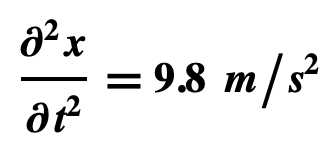• Then, using calculus, you calculate the particle’s future velocity and location from its initial velocity and location:
• Velocity at future time t is 9.8t + the initial velocity
• Location at future time t is 4.9t2 + initial location + initial velocity x t

#### Differential Geometry

• A line element is the infinitesimal distance between two neighboring points in a space or on a surface.
• Differential Geometry uses calculus on the line element to calculate geometric quantities such as lengths, areas, volumes, angles, geodesics.
• For example, the line element for two-dimensional Euclidean space in Cartesian Coordinates is: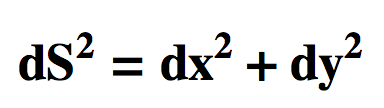• Using calculus on this line element, the lengths of lines can be calculated:

#### Tensors

• The postulates use mathematical entities called tensors: Rμν, Tμν, gμν, x𝜶, x𝜷 and x𝝁 .
• Einstein’s happiest thought was that laws of nature are universal, applying to all reference frames (coordinate systems), not just inertial frames.
• Einstein wanted to formulate the postulates of GR so they would have the same form in all reference frames.
• But he faced a problem.  He knew from Special Relativity that:
• The length of the same rod can be different in different reference frames
• The same event can take longer in one reference frame than another.
• To solve the problem Einstein used tensors, invented 20 years earlier by Gregorio Ricci-Curbastro and Tullio Levi-Civita
• A tensor is an abstract entity whose elements transform across coordinate systems.
• Tensors have upper indices (x𝜶, x𝜷, x𝝁), lower indices (Rμν, Tμν, gμν), or a combination (Fμν). Upper and lower indices refer to different kinds of transformation; so x𝜶 transforms one way across coordinate systems and x𝜶 a different way.
• A tensor with one index (x𝜶, x𝜷, x𝝁) is a vector plus transformation rules, upper or lower. A vector in 4D spacetime has one time component and three space components, e.g. (ct,x,y,z) in Cartesian coordinates and (ct,r,𝜃,𝜙) in spherical coordinates.
• A tensor with two indices (Rμν, Tμν, gμν) is a 4×4 matrix plus transformation rules.  For example, the metric tensor, gμν, has the form: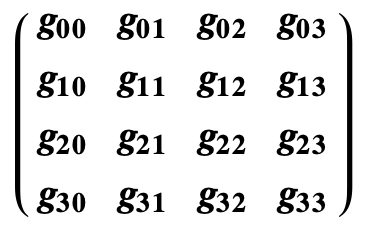• Rμν, Tμν, and gμν are symmetric tensors, meaning that six pairs of the sixteen components are duplicates, leaving ten distinct components.
• The Field Equation thus looks like this:
• It’s equivalent to ten differential equations:
• R00 – ½ R g00 = 8πG/c4 T00
• R01 – ½ R g01 = 8πG/c4 T01
• R02 – ½ R g02 = 8πG/c4 T02
• And so on.
• The Geodesic Equation uses the tensors x𝜶, x𝜷, x𝝁, called four-vectors.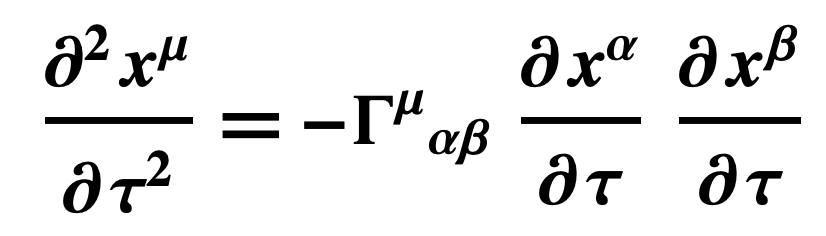• Four-vectors in General Relativity have a time component and three space components, for example
• Spacetime Cartesian coordinates:
• (ct, x, y, z)
• Spacetime Spherical Coordinates:
• (ct, r, θ, ϕ)
• The Geodesic Equation is thus equivalent to four differential equations, one for each component.  For example, using (ct, r, θ, ϕ):